Question

Aysha has 40 cubes in a bag.
18 red cubes.
13 blue cubes.
9 yellow cubes.
Aysha adds some more red cubes into the bag.
Aysha is now going to take at random a cube from the bag
The probability now that she will take a red cube is 0.5.
Work out the probability that she will take a yellow cube.

1.bonexptip

Given:

Total number of cubes = 40

Red cubes = 18

Blue cubes = 13

Yellow cubes = 9

Aysha adds some more red cubes into the bag.

The probability now that she will take a red cube is 0.5.

To find:

The probability that she will take a yellow cube.

Solution:

Let Aysha adds x red cubes into the bag. Then,

Number of total cubes = 40+x

Number of red cubes = 18+x

Probability of getting a red cube is: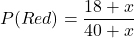The probability now that she will take a red cube is 0.5. So,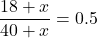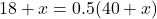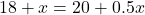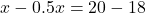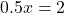Divide both sides by 0.5.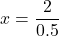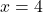It means Aysha adds 4 more red cubes into the bag.

Now, the total number of cubes int he bag is: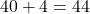Probability of getting a yellow cube is: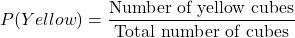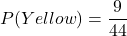Therefore, the required probability is.